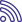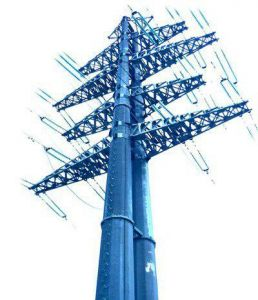Key performance indicatorsMain performance indicators
• 05.07.2023
• 2265№ Main performance indicators Unit measurements The main production indicators 01.07.2023 I Electricity production: billion kWh “-“ 1.1. In the Republic of Uzbekistan billion kWh 38,4 1.2. JSC "TPP" billion kWh 34,3 II Electricity transportation billion kWh 35,4 III Electricity export billion kWh 1,1 IV Electricity imports billion kWh 2,7

Open Data Portal of the Republic of Uzbekistan№ Main performance indicators Unit measurements The main production indicators 04.04.2023 I Electricity production: billion kWh “-“ 1.1. In the Republic of Uzbekistan billion kWh 20,1 1.2. JSC "TPP" billion kWh 18,5 II Electricity transportation billion kWh 18,8 III Electricity export billion kWh 0,8 IV Electricity imports billion kWh 1,6№ Main performance indicators Unit measurements The main production indicators for the 4 th quarter of 2022 I Electricity production: billion kWh “-“ 1.1. In the Republic of Uzbekistan billion kWh 74,3 1.2. JSC "TPP" billion kWh 66,6 II Electricity transportation billion kWh 67,0 III Electricity export billion kWh 2,7 IV Electricity imports billion kWh 5,6№ Main performance indicators Unit measurements The main production indicators for the 9 months of 2022 I Electricity production: billion kWh “-“ 1.1. In the Republic of Uzbekistan billion kWh 54,7 1.2. JSC "TPP" billion kWh 48,6 II Electricity transportation billion kWh 46,9 III Electricity export billion kWh 1,7 IV Electricity imports billion kWh 3,8№ Main performance indicators Unit measurements The main production indicators 01.07.2022 I Electricity production: billion kWh “-“ 1.1. In the Republic of Uzbekistan billion kWh 36,8 1.2. JSC "TPP" billion kWh 33,1 II Electricity transportation billion kWh 32,9 III Electricity export billion kWh 1,4 IV Electricity imports billion kWh 2,4№ Main performance indicators Unit measurements The main production indicators for the 1st quarter of 2022 I Electricity production: billion kWh “-“ 1.1. In the Republic of Uzbekistan billion kWh 19,7 1.2. JSC "TPP" billion kWh 18,5 II Electricity transportation billion kWh 17,2 III Electricity export billion kWh 0,9 IV Electricity imports billion kWh 1,6№ Main performance indicators Unit measurements The main production indicators for the 4 th quarter of 2021 I Electricity production: billion kWh “-“ 1.1. In the Republic of Uzbekistan billion kWh 71,3 1.2. JSC "TPP" billion kWh 65,6 II Electricity transportation billion kWh 63,8 III Electricity export billion kWh 2,15 IV Electricity imports billion kWh 6,2№ Main performance indicators Unit measurements The main production indicators for the 9 months of 2021 I Electricity production: billion kWh “-“ 1.1. In the Republic of Uzbekistan billion kWh 51,7 1.2. JSC "TPP" billion kWh 47,1 II Electricity transportation billion kWh 43,5 III Electricity export billion kWh 1,3 IV Electricity imports billion kWh 4,6№ Main performance indicators Unit measurements The main production indicators for the 1st half of 2021 I Electricity production: billion kWh “-“ 1.1. In the Republic of Uzbekistan billion kWh 35,0 1.2. JSC "TPP" billion kWh 32,3 II Electricity transportation billion kWh 31,2 III Electricity export billion kWh 1,2 IV Electricity imports billion kWh 3,1№ Main performance indicators Unit measurements The main production indicators for the 1 th quarter of 2021 I Electricity production: billion kWh “-“ 1.1. In the Republic of Uzbekistan billion kWh 18,3 1.2. JSC "TPP" billion kWh 17,3 II Electricity transportation billion kWh 16,1 III Electricity export billion kWh 0,8 IV Electricity imports billion kWh 2,0№ Main performance indicators Unit measurements The main production indicators for the 4 th quarter of 2020 I Electricity production: billion kWh “-“ 1.1. In the Republic of Uzbekistan billion kWh 66,4 1.2. JSC "TPP" billion kWh 60,7 II Electricity transportation billion kWh 53,9 III Electricity export billion kWh 2,7 IV Electricity imports billion kWh 5,3№ Main performance indicators Unit measurements The main production indicators for the 3 st quarter of 2020 I Electricity production: billion kWh “-“ 1.1. In the Republic of Uzbekistan billion kWh 47,8 1.2. JSC "TPP" billion kWh 43,3 II Electricity transportation billion kWh 38,2 III Electricity export billion kWh 1,8 IV Electricity imports billion kWh 3,5№ Main performance indicators Unit measurements The main production indicators for the 2 st quarter of 2020 I Electricity production: billion kWh “-“ 1.1. In the Republic of Uzbekistan billion kWh 32,2 1.2. JSC "TPP" billion kWh 29,4 II Electricity transportation billion kWh 26,5 III Electricity export billion kWh 1,2 IV Electricity imports billion kWh 2,4№ Main performance indicators Unit measurements The main production indicators for the 1st quarter of 2020 I Electricity production: billion kWh “-“ 1.1. In the Republic of Uzbekistan billion kWh 17,4 1.2. JSC "TPP" billion kWh 16,1 II Electricity transportation billion kWh 14,0 III Electricity export billion kWh 0,85 IV Electricity imports billion kWh 1,9№ Main performance indicators Unit measurements The main production indicators for 9 months of 2019 I Electricity production: billion kWh “-“ 1.1. In the Republic of Uzbekistan billion kWh 46,2 1.2. JSC "TPP" billion kWh 40,6 II Electricity transportation billion kWh 36,1 III Electricity export billion kWh 1,3 IV Electricity imports billion kWh 2,6

>